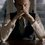# Need help in "Differentiation under integral sign"!

Well, I was doing some problems where I could use the technique mentioned above. But I was so much confused about the constant we have to add at the end. In most of the problems, the constant was turning out to be the answer so I couldn't work out a guess even. Can anyone please help? I checked out Wikipedia and they say "By definition $I(\text{something}) = \text{something else}$" -_- How does one know the definition of integral? Kindly enlighten me!Note by Kartik Sharma
6 years, 2 months ago

This discussion board is a place to discuss our Daily Challenges and the math and science related to those challenges. Explanations are more than just a solution — they should explain the steps and thinking strategies that you used to obtain the solution. Comments should further the discussion of math and science.

When posting on Brilliant:

• Use the emojis to react to an explanation, whether you're congratulating a job well done , or just really confused .
• Ask specific questions about the challenge or the steps in somebody's explanation. Well-posed questions can add a lot to the discussion, but posting "I don't understand!" doesn't help anyone.
• Try to contribute something new to the discussion, whether it is an extension, generalization or other idea related to the challenge.

MarkdownAppears as
*italics* or _italics_ italics
**bold** or __bold__ bold
- bulleted- list
• bulleted
• list
1. numbered2. list
1. numbered
2. list
Note: you must add a full line of space before and after lists for them to show up correctly
paragraph 1paragraph 2

paragraph 1

paragraph 2

[example link](https://brilliant.org)example link
> This is a quote
This is a quote
    # I indented these lines
# 4 spaces, and now they show
# up as a code block.

print "hello world"
# I indented these lines
# 4 spaces, and now they show
# up as a code block.

print "hello world"
MathAppears as
Remember to wrap math in $$ ... $$ or $ ... $ to ensure proper formatting.
2 \times 3 $2 \times 3$
2^{34} $2^{34}$
a_{i-1} $a_{i-1}$
\frac{2}{3} $\frac{2}{3}$
\sqrt{2} $\sqrt{2}$
\sum_{i=1}^3 $\sum_{i=1}^3$
\sin \theta $\sin \theta$
\boxed{123} $\boxed{123}$

Sort by:

- 6 years, 2 months ago

Can you please state your question more clearly? Maybe you can tell us one of the problems which confused you?

I hope the following is of some help:

Consider a known function $f(x)$. I think you may be familiar with the concept of derivative w.r.t $x$ of $f(x)$. This is simply the rate of change of $f$ w.r.t $x$ for some $x$.

Now, on the same lines... why don't we think backwards? Given $f(x)$, can we find a function $g(x)$ such that the derivative of $g(x)$ w.r.t $x$ is same as $f(x)$? The answer to this question is yes, and it is possible through integration. This means:

$\frac { d }{ dx } (g\left( x \right) )=f\left( x \right)$ or:

$g\left( x \right) =\int { f\left( x \right) dx }$

There are different methods that we can use to evaluate such integrals. But here, we will stick to discussing the significance of the constant.

If we observe, the answer to our question is satisfied by infinitely many $g(x)$, each of which differ from one another by a constant. This is due to the fact that while differentiating $g(x)$, the constant term vanishes and hence, to get the whole set of functions which on differentiating yield $f(x)$, we add a constant to the integral after integrating.

- 6 years, 2 months ago

You totally misunderstood my question. "Differentiation under integral sign" is a technique used to integrate various integrals made famous particularly by Feynman. Check out this! I must have shared the link previously only. When we integrate $I'(\alpha)$, we have to add a constant of integration. Now, my question is how can we get that constant.

- 6 years, 2 months ago

Kartik as in differential equations the value of constant is found out using the initial values. As an Eg.:

You have f(y) +C= Integral of g(x) with respect to x from x=0 to x=y.

It is easy to observe that f(0)=0

With this initial value you can find the constant .

- 6 years, 2 months ago

Hmm okay now I see! Thanks! BTW, I was doing your problems only when I got this doubt!

- 6 years, 2 months ago

But if it is like

f(y) + C = integral of g(x,y) w.r.t x from x =0 to x = a

for any constant a.

Then, what can we do?

- 6 years, 2 months ago

For example, check out Example 5 from the Wiki page. There they say that $I(\pi/2) = 0$ by definition, what does that mean?

- 6 years, 2 months ago

They say that it is zero by definition because the integrand becomes zero everywhere if we put $\alpha= \pi/2$.

- 6 years, 2 months ago

Try to put a value of y such that g(x,y)=0

Eg, g(x,y)= e^(-y).sin(x) , put y= infinity.

- 6 years, 2 months ago

Hmm, okay I will try! Thanks!

- 6 years, 2 months ago